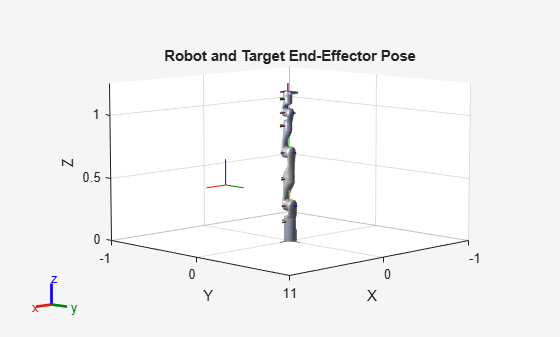Model rigid body tree motion given task-space reference inputs

## Description

The `taskSpaceMotionModel` object models the closed-loop task-space motion of a manipulator, specified as a rigid body tree object. The motion model behavior is defined by the MotionType property.

## Creation

### Syntax

``motionModel = taskSpaceMotionModel``
``motionModel = taskSpaceMotionModel("RigidBodyTree",tree)``
``motionModel = taskSpaceMotionControlModel(Name,Value)``

### Description

````motionModel = taskSpaceMotionModel` creates a motion model for a default two-joint manipulator.```
````motionModel = taskSpaceMotionModel("RigidBodyTree",tree)` creates a motion model for the specified `rigidBodyTree` object.```

example

````motionModel = taskSpaceMotionControlModel(Name,Value)` sets additional properties specified as name-value pairs. You can specify multiple properties in any order.```

## Properties

expand all

Rigid body tree robot model, specified as a `rigidBodyTree` object that defines the inertial and kinematic properties of the manipulator.

This property defines the body that will be used as the end effector, and for which the task space motion is defined. The property must correspond to a body name in the `rigidBodyTree` object of the RigidBodyTree property. If the rigid body tree is updated without also updating the end effector, the body with the highest index becomes the end-effector body by default.

Proportional gain for PD control, specified as a 6-by-6 matrix.

Derivative gain for proportional-derivative (PD) control, specified as a 6-by-6 matrix.

Damping ratios on each joint, specified as a scalar or n-element vector, where n is the number of nonfixed joints in the `rigidBodyTree` object in the RigidBodyTree property. If a scalar is specified, then `DampingRatio` becomes an n-element vector of value `s`, where `s` is the specified scalar

Type of motion, specified as `"PDControl"`, which uses proportional-derivative (PD) control mapped to the joints via a Jacobian-Transpose controller. The control is based on the specified Kp and Kd properties.

## Object Functions

 `derivative` Time derivative of manipulator model states `updateErrorDynamicsFromStep` Update values of NaturalFrequency and DampingRatio properties given desired step response

## Examples

collapse all

This example shows how to create and use a `taskSpaceMotionModel` object for a manipulator robot arm in task-space.

Create the Robot

`robot = loadrobot("kinovaGen3","DataFormat","column","Gravity",[0 0 -9.81]);`

Set Up the Simulation

Set the time span to be 1 second with a timestep size of 0.02 seconds. Set the initial state to the home configuration of the robot, with a velocity of zero.

```tspan = 0:0.02:1; initialState = [homeConfiguration(robot);zeros(7,1)];```

Define a reference state with a target position and zero velocity.

```refPose = trvec2tform([0.6 -.1 0.5]); refVel = zeros(6,1);```

Create the Motion Model

Model the behavior as a system under proportional-derivative (PD) control.

`motionModel = taskSpaceMotionModel("RigidBodyTree",robot,"EndEffectorName","EndEffector_Link");`

Simulate the Robot

Simulate the behavior over 1 second using a stiff solver to more efficiently capture the robot dynamics`.` Using `ode15s` enables higher precision around the areas with a high rate of change.

`[t,robotState] = ode15s(@(t,state)derivative(motionModel,state,refPose,refVel),tspan,initialState);`

Plot the Response

Plot the robot's initial position and mark the target with an X.

```figure show(robot,initialState(1:7)); hold all plot3(refPose(1,4),refPose(2,4),refPose(3,4),"x","MarkerSize",20)```

Observe the response by plotting the robot in a 5 Hz loop.

```r = rateControl(5); for i = 1:size(robotState,1) show(robot,robotState(i,1:7)',"PreservePlot",false); waitfor(r); end```Craig, John J. Introduction to Robotics: Mechanics and Control. Upper Saddle River, NJ: Pearson Education, 2005.

 Spong, Mark W., Seth Hutchinson, and Mathukumalli Vidyasagar. Robot Modeling and Control. Hoboken, NJ: Wiley, 2006.

##### Support평가판 신청